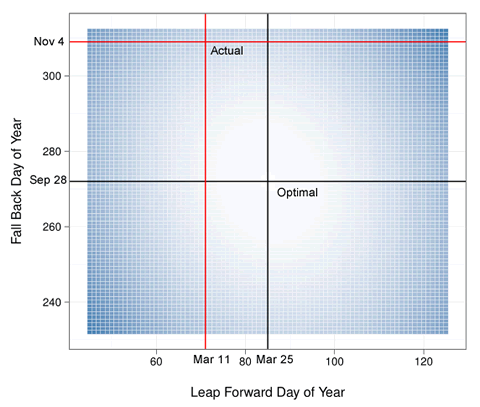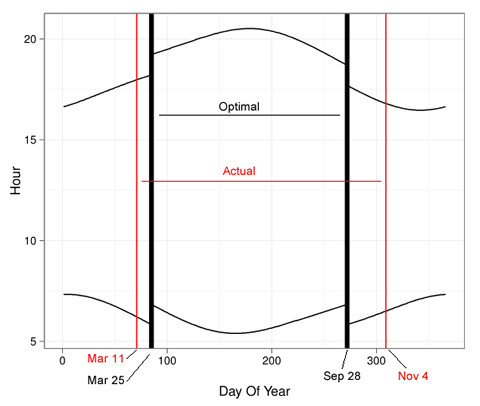Main

## Follow-up: So … daylight savings time does not minimize variance in sunrises

Filed in Encyclopedia ,Ideas ,RSubscribe to Decision Science News by Email (one email per week, easy unsubscribe)

NOT SURE WHY DAYLIGHT SAVINGS TIME IS WHEN IT ISLast week we posted a nice theory about daylight savings time, in particular, that its dates were chosen to reduce variance in the time of sunrise. It looked plausible from the graph.

We were talking to our Microsoft Research colleague Jake Hofman who suggested “why don’t you just find the optimal dates to change the clock by one hour?” So we did. We got the times of sunrise for New York City from here, threw them into R, and optimized.

The result was surprising. The dates of daylight savings time do not come close to minimizing variance in sunrise. If they did, in 2012, DSL would have started on March 25th and ended on September 28th. In actuality, it started on March 11th and ended on November 4th. For NYC, daylight savings time starts too early and ends too late to minimize variance in sunrise. In the heatmap above, the higher the variance, the bluer the squares. The variance minimizing dates are shown in black, and the actual ones in red. The same color coding is used in the plot below, which also shows how the hours would shift if the variance minimizing dates were chosen (see last week’s post for how they actually change).So what then is the logic behind DSL? We’re not quite sure. There are some leads in this article. We also learned that the US lengthened DSL in 2007 as it believes it that DSL saves energy, but it is not clear that it does.

If you want to play with this, the data are here: Sunrise and Sunset data for New York City in 2012. The source of the data is here.

``` library(ggplot2) #data from http://aa.usno.navy.mil/data/docs/RS_OneYear.php setwd("C:/Dropbox/Projects/Sunrise/") df=read.table("nyc_sunrise.txt",colClasses="character") temp_times=unlist(df[,c(paste("V",seq(2,24,2),sep=""), paste("V",seq(3,25,2),sep=""))]) l=length(temp_times)/2 df=data.frame(day=1:31,stime=temp_times, sun=c(rep("rise",l),rep("set",l))) rm(temp_times,l) hour=as.numeric(substr(df\$stime,1,2)) minute=as.numeric(substr(df\$stime,3,4))/60 df\$time=hour+minute df=subset(df,!is.na(df\$time)) df\$day_of_year=1:(nrow(df)/2) p=ggplot(data=subset(df,sun=="rise"),aes(x=day_of_year,y=time)) p=p+geom_line() p=p+geom_line(data=subset(df,sun=="set"),aes(x=day_of_year,y=time)) p zerovec=function(i,j){ c(rep(0,i-1), rep(1,j-i+1), rep(0,len-j))} currvec=df[df\$sun=="rise","time"] len=length(currvec) get_var=function(i,j) { var(currvec + zerovec(i,j))} vget_var=Vectorize(get_var) result=expand.grid(spring_forward=45:125,fall_back=232:312) result=subset(result,spring_forward<fall_back) result\$var=with(result,vget_var(spring_forward,fall_back)) resout=result[which.min(result\$var),] resout #Heatmap p =ggplot(data=result, aes(spring_forward, fall_back)) + geom_tile(aes(fill = var), colour = "white") + scale_fill_gradient(low = "white", high = "steelblue") p=p+geom_vline(xintercept=as.numeric(resout))+ geom_hline(yintercept=as.numeric(resout)) p=p+geom_vline(xintercept=71,color="red")+ geom_hline(yintercept=309,color="red") p=p+ylab("Fall Back Day of Year\n")+theme_bw() p=p+xlab("\nLeap Forward Day of Year")+opts(legend.position="none") p ggsave("heatmap.pdf",p,width=6) #For 2012, optimal spring forward day (85)is 03/25/2012 #For 2012, optimal fall back day (272) is 09/28/2012 #Actual DSL start was 3/11/2012 (71) #Actual DSL end was 11/4/2012 (309) p=ggplot(data=subset(df,sun=="rise"), aes(x=day_of_year,y=time+zerovec(85,272))) p=p+geom_line() p=p+geom_line(data=subset(df,sun=="set"), aes(x=day_of_year,y=time+zerovec(85,272))) p=p+geom_vline(xintercept=85,lwd=2)+ geom_vline(xintercept=272,lwd=2) p=p+geom_vline(xintercept=71,color="red")+ geom_vline(xintercept=309,color="red") p=p+ylab("Hour")+theme_bw() p=p+xlab("\nDay Of Year")+opts(legend.position="none") p ggsave("timeshift.pdf",p,width=6) ```

Figures created with Hadley Wickham’s ggplot2

## 5 Comments

1. Philip says:

Wasn’t the previous graph for Chicago rather than NYC? Maybe run on all cities and see for which city it is close to optimal.

December 3, 2012 @ 9:33 am

2. dan says:

Indeed, the other post was for Chicago. Doing all cities is a good idea, but I’ve wasted enough time on this already 🙂

December 3, 2012 @ 10:31 am

3. Anonymous says:

I always thought that the start of spring was important i.e. the measurement unit for this optimization was season instead of day.

In Iran where they use Persian calendar, DLS starts at March 21 or 22 and ends in September 22 or 23 (depending on whether the Persian new year started on March 20 Or 21.)

December 3, 2012 @ 8:32 pm

4. Carl Witthoft says:

On the other hand, that foolish change in the start/end of DLS in the USA led to a massive code rewrite for clocks inside other machines — and the immediate obsolescence of clocks and watches with internal ROM code to change from DLS to standard time.

December 4, 2012 @ 1:12 pm

5. Rohit Gupta says:

I tested the data for San Jose in California. There is a little variation as compared to NYC. The start time is still March 25th, the end time however comes out to be Sep 30th for 2012 for minimum variance.

One thing that’s clear is if we keep changing our clocks continuously instead of once in an year, we will be able to bring down the variance in the time of sunrise to zero! This was probably the science behind the use of sundial. Every time the sun rises, the sundial would show the same time (based on the shadow) irrespective of what time our modern atomic clocks shows. It would be fun to have a clock that automatically adjusts to morning sunrise everyday. Everyday variations will be small enough to have any effect on our sleep cycle and we would have same sunrise everyday!

December 4, 2012 @ 11:22 pm

### Write Comment

XHTML: <a href="" title=""> <abbr title=""> <acronym title=""> <b> <blockquote cite=""> <cite> <code> <del datetime=""> <em> <i> <q cite=""> <s> <strike> <strong>